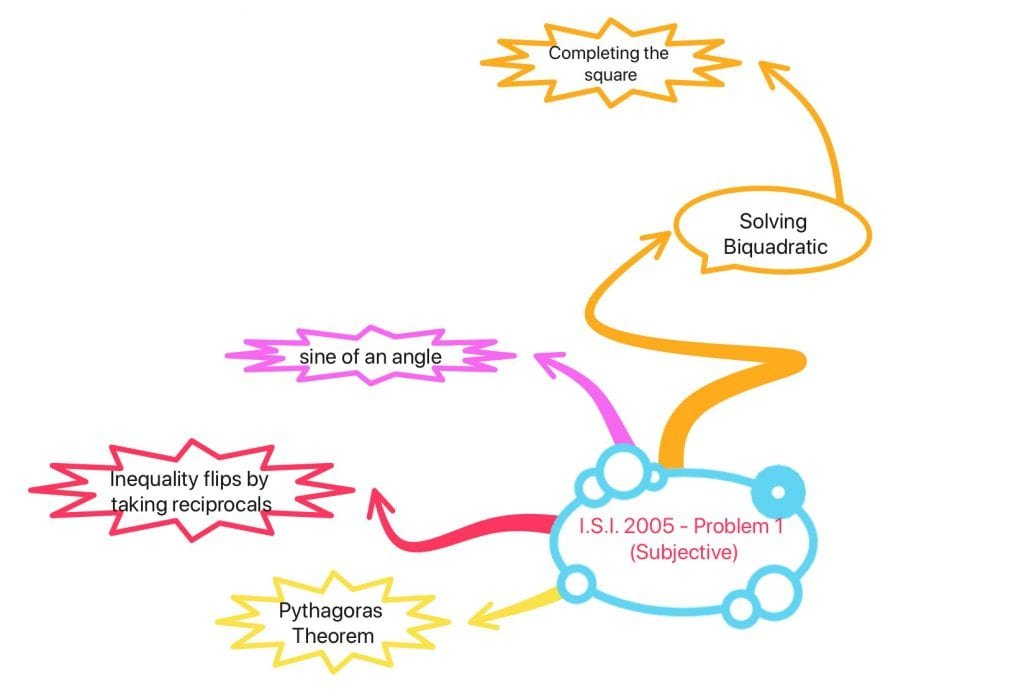How Cheenta works to ensure student success?
Explore the Back-Story

# An interesting biquadratic from ISI Entrance 2005Problem

Let $a,b$ and $c$ be the sides of a right angled triangle. Let $\displaystyle{\theta }$ be the smallest angle of this triangle. If $\displaystyle{ \frac{1}{a}, \frac{1}{b} }$ and $\displaystyle{ \frac{1}{c} }$ are also the sides of a right angled triangle then show that $\displaystyle{ \sin\theta=\frac{\sqrt{5}-1}{2}}$

Topic

Main Idea

It is usually hard to solve biquadratic equations. However, sometimes, combining tools from geometry and strategies like completing the square it is possible to do so.

Source

I.S.I. B.Stat. Entrance 2005, Subject Problem 1

Suggested Book

Challenges and Thrills in Pre College Mathematics
Excursion Of Mathematics

Watch video

Knowledge graph.

Problem

Let $a,b$ and $c$ be the sides of a right angled triangle. Let $\displaystyle{\theta }$ be the smallest angle of this triangle. If $\displaystyle{ \frac{1}{a}, \frac{1}{b} }$ and $\displaystyle{ \frac{1}{c} }$ are also the sides of a right angled triangle then show that $\displaystyle{ \sin\theta=\frac{\sqrt{5}-1}{2}}$

Topic

Main Idea

It is usually hard to solve biquadratic equations. However, sometimes, combining tools from geometry and strategies like completing the square it is possible to do so.

Source

I.S.I. B.Stat. Entrance 2005, Subject Problem 1

Suggested Book

Challenges and Thrills in Pre College Mathematics
Excursion Of Mathematics

Watch video

Knowledge graph.

This site uses Akismet to reduce spam. Learn how your comment data is processed.

### Knowledge Partner